# Use of Polystyrene in Ribbed Slabs: Structural Design Example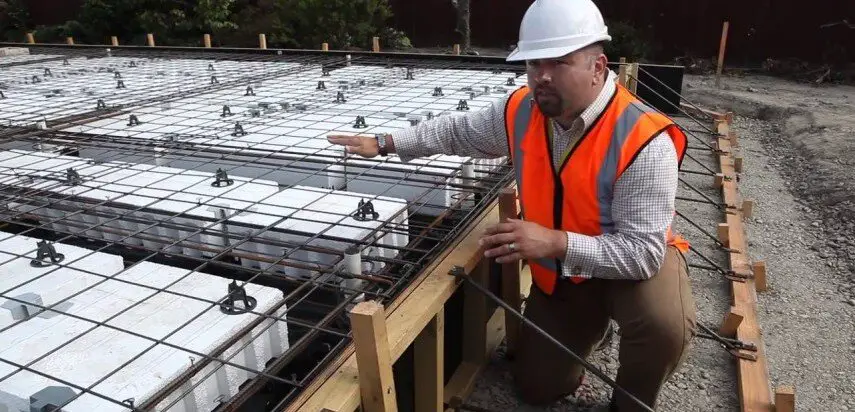For long span slabs in a building subjected to light load, ribbed slabs are more economical than solid slabs. In ribbed slab construction, reduction in volume of concrete  is achieved by removing some of the concrete below the neutral axis of the section, under the assumption that the tensile strength of concrete is negligible. Two major forms of construction of ribbed slab are;

(1) Ribbed slab without permanent blocks
(2) Ribbed slab with permanent blocks

The major advantage of ribbed slab with permanent blocks is the reduction in the quantity of formwork required, given  the fact that strength of the blocks is normally not assumed to contribute to the strength of the slab in design. In Nigeria, clay hollow pot and sandcrete blocks have been successfully used, but on the rise is the polystyrene. Polystyrene offers the advantage of light weight, improved fire resistance, and economy, at the expense of less rigidity compared to clay hollow pot or blocks.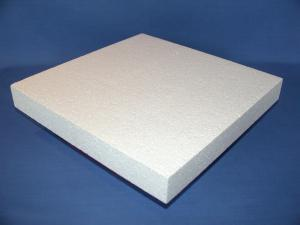Fig 2: Expanded Polystyrene (EPS)

In a generic sense, the use of polystyrene is to encourage savings in formwork costs, and may not be assumed to contribute to the strength of the floor in any way.

In this post, we are going to consider the design of a floor (see figure 2) subjected to the following loading conditions;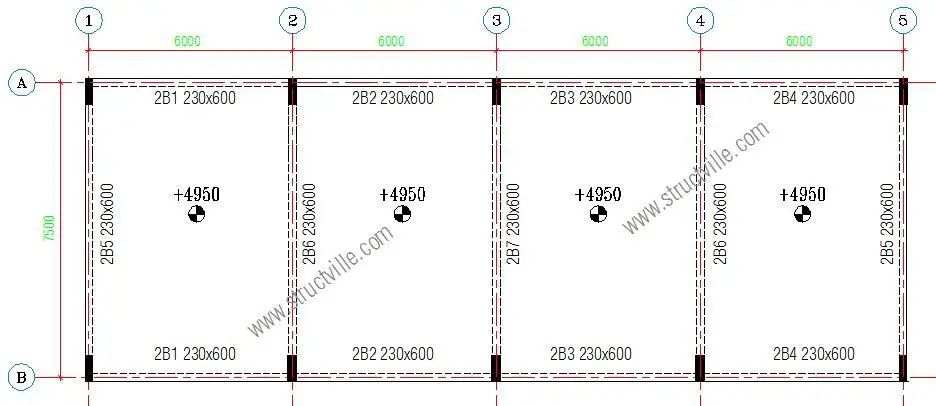Fig 3: Preliminary Structural Arrangement

Finishes – 1.2 kN/m2
Partition allowance – 1.5 kN/m2

fck = 30 Mpa, fyk = 500 Mpa, Concrete cover = 25 mm

The structural engineer decided the scheme the structure as shown in figure 4. The slab is made thick close to the supports, to enable adequate transmission of shear forces.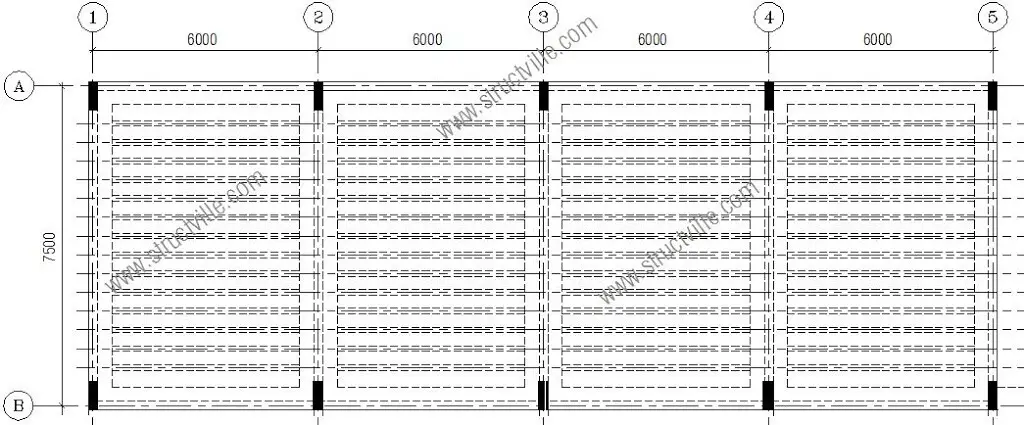Fig 4: Adopted Structural Scheme

From basic span/effective depth ratio of 26, let us choose a trial depth;
6000/26 = 231 mm

Let us try a total depth of 250 mmFig 5: Transverse Section Through The Slab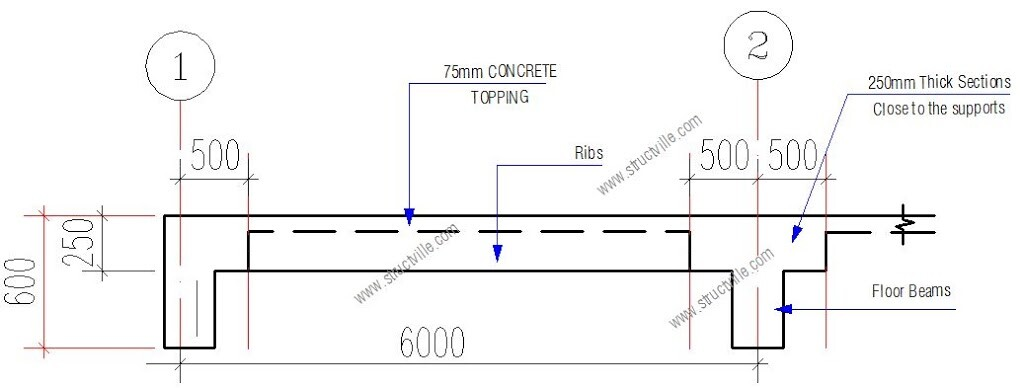Fig 6: Longitudinal Section Through the Slab

For 500mm c/c rib spacing;

Permanent Actions
Weight of topping: 0.075 × 25 × 0.5 = 0.9375 kN/m
Weight of ribs: 0.15 × 0.175 × 25 = 0.656 kN/m
Weight of finishes: 1.2 × 0.5 = 0.6 kN/m
Partition allowance: 1.5 × 0.5 = 0.75 kN/m
Weight of heavy duty EPS (16 kg/m3) = 0.156 × 0.175 × 0.5 = 0.01365 kN/m

Variables Action(s)
Occupancy load qk: 2.5 × 0.5 = 1.25 kN/m

At ultimate limit state; 1.35gk + 1.5qk = 1.35(2.95) + 1.5(1.25) = 5.8575 kN/m

Structural Analysis
The design load per span at ultimate limit state (F) is given by = 5.8575 kN/m × 6m = 35.145 kN/m

From clause 3.5.2.3 of BS 8110-1:1997, we can use the simplified method for structural analysis.

At the middle of span 1-2; M = 0.075 × 35.145 × 6 = 15.815 kNm
At support 2, MEd = -0.086 × 35.145 × 6 = 18.134 kNm

Designing the span as a T-beam;
MEd = 15.815 kN.m

Effective depth (d) = h – Cnom – ϕ/2 – ϕlinks
Assuming ϕ12 mm bars will be employed for the main bars, and ϕ10 mm bars for the stirrups (links)
d = 250 – 25 – (12/2) -10 = 209 mm

k = MEd/(fckbd2) = (15.815 × 106)/(30 × 500 × 2092) = 0.024
Since k < 0.167, no compression reinforcement required

z = d[0.5+ √(0.25 – 0.882k)]
k’ = 0.024
z = d[0.5+ √((0.25 – 0.882(0.024))] = 0.95d = 198.55 mm

Depth to neutral axis x = 2.5(d – z) = 2.5(209 – 198.55) = 26.125 mm < 1.25hf (93.75mm)
Therefore, we design rib as a rectangular section

Area of tension reinforcement As1 = MEd / (0.87fyk z)
As1 = MEd / (0.87fyk z) = (15.815 × 106) / (0.87 × 500 × 0.95 × 209) = 183 mm2

Provide 2H12  Bot (ASprov = 226 mm2)

Check for deflection
ρ = As,req /bd = 183 / (500 × 209) = 0.00175
ρ0 = reference reinforcement ratio = 10-3√(fck) = 10-3√(30) = 0.00547
Since  ρ ≤ ρ0;
L/d = k [11 + 1.5√(fck) ρ0/ρ + 3.2√(fck) (ρ0 / ρ – 1)(3⁄2)]
k = 1.3
L/d = 1.3 [11 + 1.5√(30) × (0.00547/0.00175) + 3.2√(30) × [(0.00547 / 0.00175) – 1](3⁄2)]
L/d = 1.3[11 + 25.68 + 54.321] = 118.3

βs = (500 Asprov)/(fyk Asreq) = (500 × 226) / (500 × 183) = 1.23

beff/bw = 500/150 = 3.33

Therefore multiply basic length/effective depth ratio by 0.8

Therefore limiting L/d = 1.23 × 0.8  × 118.3 = 116.41
Actual L/d = 6000/209 = 28.708

Since Actual L/d (28.708) < Limiting L/d (116.41), deflection is satisfactory.

Design of Support 2;
MEd = 18.134 kN.m

Effective depth (d) = h – Cnom – ϕ/2 – ϕlinks
Assuming ϕ12 mm bars will be employed for the main bars, and ϕ10 mm bars for the stirrups (links)
d = 250 – 25 – (12/2) -10 = 209 mm

k = MEd/(fckbd2) = (18.134 × 106)/(30 × 150 × 2092) = 0.0922
Since k < 0.167, no compression reinforcement required

z = d[0.5+ √(0.25 – 0.882k)]
k = 0.092
z = d[0.5+ √((0.25 – 0.882(0.092))] = 0.91d

Area of tension reinforcement As1 = MEd / (0.87fyk z)
As1 = MEd / (0.87fyk z) = (18.134 × 106) / (0.87 × 500 × 0.91 × 209) = 219 mm2
Provide 2H12  Top (ASprov = 226 mm2)

Shear Design
Maximum shear force in the rib VEd = 0.6F = 0.6 × 35.145 = 21.087 kN

VRd,c = [CRd,c.k.(100ρ1 fck)(1/3) + k1cp]bw.d ≥ (Vmin + k1cp) bw.d

CRd,c = 0.18/γc = 0.18/1.5 = 0.12
k = 1 + √(200/d) = 1 + √(200/209) = 1.97 < 2.0, therefore, k = 1.97
Vmin = 0.035k(3/2) fck0.5
Vmin = 0.035 × (1.97)1.5 × 300.5 = 0.53 N/mm2
ρ1 = As/bd = 226/(150 × 209) = 0.0072 < 0.02; Therefore take 0.0072

VRd,c = [0.12 × 1.97 (100 × 0.0072 × 30 )(1/3)] × 150 × 209 = 20639.67 N = 20.639 kN

Since VRd,c (20.639 kN) < VEd (21.087 kN), shear reinforcement is required.
The compression capacity of the compression strut (VRd,max) assuming θ = 21.8° (cot θ = 2.5)

VRd,max = (bw.z.v1.fcd) / (cot⁡θ + tanθ)
V1 = 0.6(1 – fck/250) = 0.6(1 – 30/250) = 0.528
fcd = (αcc fck) / γc = (0.85 × 30) / 1.5 = 17 N/mm2
Let z = 0.9d

VRd,max = [(150 × 0.9 × 209 × 0.528 × 17) / (2.5 + 0.4)] × 10-3 = 87.333 kN

Since < VEd < VRd,c < VRd,max

Hence Asw / S = VEd / (0.87 Fyk z cot θ) = 21087 / (0.87 × 500 × 0.9 × 209 × 2.5 ) = 0.103

Minimum shear reinforcement;
Asw / S = ρw,min × bw × sinα (α = 90° for vertical links)
ρw,min = (0.08 × √(fck)) / fyk = (0.08 × √30) / 500 = 0.000876
Asw/Smin = 0.000876 × 150 × 1 = 0.131
Maximum spacing of shear links = 0.75d = 0.75 × 209 = 156.75 mm

Provide H8mm @ 150 mm c/c as shear links.

Slab Topping
A142 BRC Mesh can be provided or H8 @ 250mm c/c

For more information on design and consultancy to accomplish the most challenging design brief, contact the author on info@structville.com. Thank you, and God bless you.

1.Taofeek Adegbite

Good job!

2.relu59

Thank you very much. !

3.Arc. Nwachukwu Bethel

Please is it possible to have a slab of 9.9m width by 52.4m that will carry another slab and partitions up with out any supporting columns aside from the ones on the perimeter walls.

•Ubani Obinna

Yes it is possible.